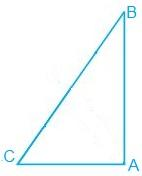Newbie

# ABC is a right-angled triangle in which A = 90° and AB = AC. Find B and C. Q.7

• 0

Sir please help me to solve the ncert class 9th solution of chapter triangles.How I solve this question of exercise 7.2 question number 7. Find the simplest and easiest solution of this question , also give me the best solution of this question.ABC is a right-angled triangle in which A = 90° and AB = AC. Find B and C

Share

1.In the question, it is given that

A = 90° and AB = AC

AB = AC

⇒ B = C (They are angles opposite to the equal sides and so, they are equal)

Now,

A+B+C = 180° (Since the sum of the interior angles of the triangle)

∴ 90° + 2B = 180°

⇒ 2B = 90°

⇒ B = 45°

So, B = C = 45°

• 0